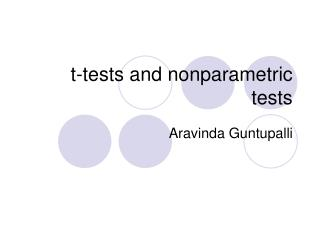DownloadDownload Presentationt-tests and nonparametric tests

t-tests and nonparametric tests

Télécharger la présentationt-tests and nonparametric tests

- - - - - - - - - - - - - - - - - - - - - - - - - - - E N D - - - - - - - - - - - - - - - - - - - - - - - - - - -
Presentation Transcript

1. t-tests and nonparametric tests Aravinda Guntupalli

2. t-tests • Different t-tests are used for independent subjects, or matched subjects/repeated measures. • The basic principle of t-tests is to compare differences in group means in order to make inferences about the wider population from which they are drawn. • one sample t-test • two independent samples t-test • Paired samples t-test

3. One sample t-test • Sample mean – population mean Standard error A one sample t-test is used to test the hypothesis that a sample is drawn from a population with a known mean but an unknown standard deviation

4. Two independent samples t-test (1) • A two independent samples t-test compares the difference between the means of the two variables. • Samples are called independent if there is no relationship between the cases described by the different groups. • To express this in another way, two random from a wider population (eg. of men and women) are by definition independent samples because the selection of one group of cases does not influence the selection of another group of cases.

5. Two independent samples t-test (2) • This approach tests the null hypothesis that two population means are equal based on the results observed in two independent samples. Sample 1 mean – sample 2 mean Standard error of the difference in means

6. Paired t-tests • Groups in a paired sample design are not independent because the same people are in both groups (eg. pre- and post-test). • In this case we are testing whether the average difference between a pair of (related) measures is significantly different from zero.

7. When to use paired t-test? • If we are using an quasi-experimental design to evaluate the effectiveness of a particular intervention we should use the paired samples t-test (where our data are normally distributed). • The null hypothesis (H0) for a paired sample t-test is that there is no difference between the mean values for the two members of a pair in the population (eg. case x at event a and event b). • The alternative hypothesis (H1) is that there is a difference in the mean values.

8. Anova • In most studies in the social sciences, we collect information about more than two variables and we often want to examine these simultaneously. • For this, we need to do a multivariate analysis. Despite it’s name, ANOVA, like the t-test is concerned with the testing of hypotheses about means. • It uses the F test to check whether the null hypothesis, that several population means are equal can be either rejected or not.

9. Non-parametric statistics

10. Why non-parametric ? • In many situations in the social sciences the properties of the data we are investigating mean that parametric methods are not appropriate. • In these situations we need to apply non-parametric, or ‘distribution-free’, methods to our data in order to address key research hypotheses.

11. Example • One hundred random samples of 100 adults each are drawn from the general population and the mean height in each sample is computed. • The distribution of mean scores across samples will most likely approximate the normal distribution. • In the above example it is assumed that in the underlying population from which our samples are derived the variable of interest (eg. height) is normally distributed (ie. it is characterised by a bell-shape distribution).

12. Deviation from normal distribution • However, in the social sciences we frequently encounter situations where there are serious departures from these distributional assumptions. • Some times the sample is not large enough to assume the normality.

13. What about categorical variables? • Finally, statistical tests based upon normality assumptions usually assume a high degree of measurement precision, so that variables are measured on an interval scale. • This assumption is frequently violated in the social sciences where we are often working with ‘cruder’ nominal and ordinal level data. • In these conditions we need to use tests that make no assumptions about the underlying distribution of the population – distribution-free or nonparametric statistics.

14. Which one to choose? • There is often more than one nonparametric equivalent for each parametric general type of test. • Tests of difference between groups: independent samples – Mann Whitney U test • Tests of difference between variables: dependent samples - Wilcoxon's matched pairs test • Tests of relationships between variables – Chi Square tests, Spearman’s Rho

15. Thank You